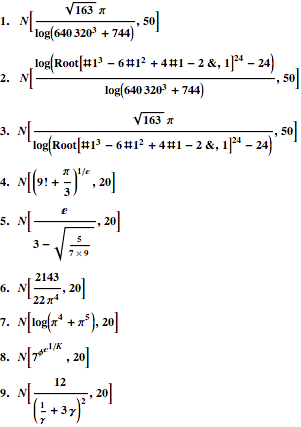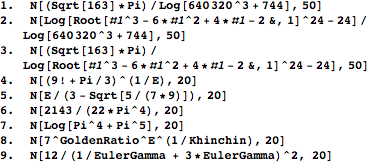Browse by Topic
Related Topics

# 9–9–9

Number 9, number 9, number 9.

The Beatles’ “Revolution 9” has the above loop, and their version of Rock Band is being released today. The movie 9 comes out today, too.

When a number has a lot of nines in it, like .99999999999999999, many computer systems can run into rounding problems. Fortunately, Mathematica can handle both exact and numeric forms. Here are exact forms of various
numbers whose numeric forms have lots of nines.Can your system figure these numbers out? Here are the Mathematica input forms for them:Numbers such as these occur in the study of almost integers. When trigonometric functions are added, then the number of nines can greatly increase. For example, 2017 21/5/π ≈ 737.50000000208, and thus sin(2017 21/5) ≈ –0.99999999999999997857. Pisot numbers can also be fantastically close to an integer.

Here are the numerical approximations for the numbers above.

1.   0.99999999999999999999999999999992878288974707564089
2.   0.99999999999999999999999999999992888272478918067295
3.   0.99999999999999999999999999999999990016495789496794
4.   110.99999996188658332
5.   0.99999996813007188185
6.   0.99999999871766046865
7.   5.9999999561918933296
8.   49.999999106159879944
9.   0.99999994563238375162

As the Beatles might say, “Take this, brother; may it serve you well…. Number 9, number 9, number 9.”